# Density estimation

21 March 2019

$\newcommand{\Expect}{\mathbb{E}\left[ #1 \right]} \newcommand{\Indicator}{\mathbb{1}\left( #1 \right)}$

# Data: body weights of cats

library(MASS)
data(cats)
head(cats$Bwt) ##  2.0 2.0 2.0 2.1 2.1 2.1 summary(cats$Bwt)
##    Min. 1st Qu.  Median    Mean 3rd Qu.    Max.
##   2.000   2.300   2.700   2.724   3.025   3.900

# The empirical cumulative distribution function

• ECDF: $\hat{F}_n(a) = \frac{1}{n}\sum_{i=1}^{n}{\Indicator{x_i \leq a}}$
• Makes a jump at each distinct value seen in the data
• Constant in between the jumps

plot(ecdf(cats$Bwt), xlab = "Body weight (kg)", ylab = "Cumulative probability")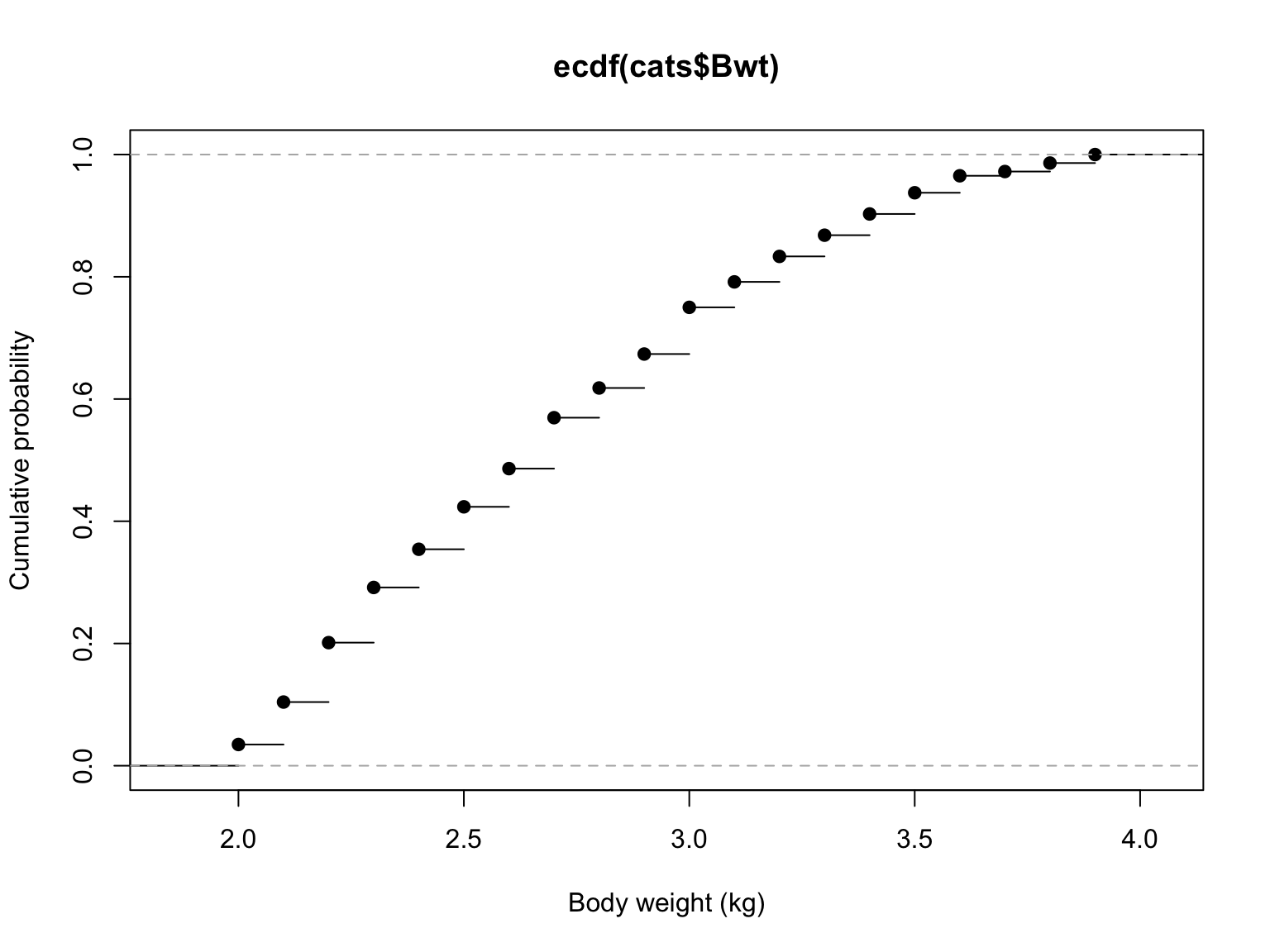# The ECDF estimates the CDF • Suppose $$x_1, x_2, \ldots x_n$$ are IID with CDF $$F$$. Then for any $$a$$, $\hat{F}_n(a) \rightarrow F(a)$ by law of large numbers • Also: see backup slides # The CDF is not enough• What’s the probability of a cat weighing between $$3.21$$ and $$3.22$$ kg? • ECDF says: exactly 0 • Q from discussion: What about running a smoother (e.g., a spline) through the ECDF? • A: You could do that, but you’d need to make sure the curve was always monotone, between 0 and 1, etc. • Once we estimate the density, we can recover the CDF by integrating # The CDF is not enough • More generally, pdf is derivative of CDF, $$f(x) = dF/dx$$ • Derivative of an empirical CDF is either 0 or $$\infty$$ • Where is it $$\infty$$? • How do we estimate a pdf? # What about a histogram? hist(cats$Bwt, xlab = "Body weight (kg)", ylab = "Number of cats", breaks = 20)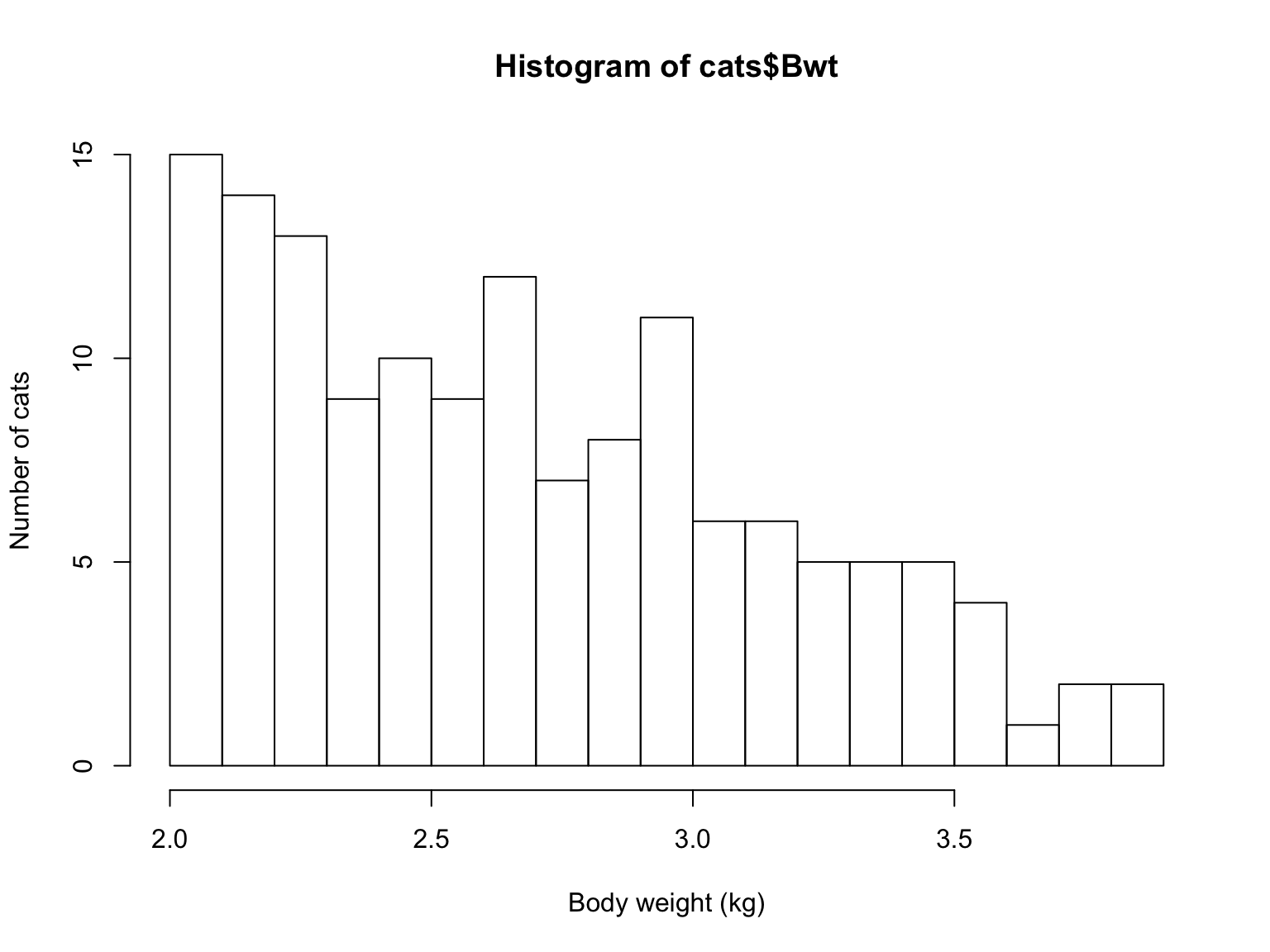• Gives us an idea of where we have more or fewer data points…

# Fitting a parametric model

• Pick a parametric pdf that looks sensible
• For example, the gamma distribution with shape parameter $$a$$ and scale $$s$$ is $f(x) = \frac{1}{s^a \Gamma(a)} x^{a-1} e^{-x/s}$
• Exponential is the special case $$a=1$$
• $$a > 1$$ is a hump with an exponential right tail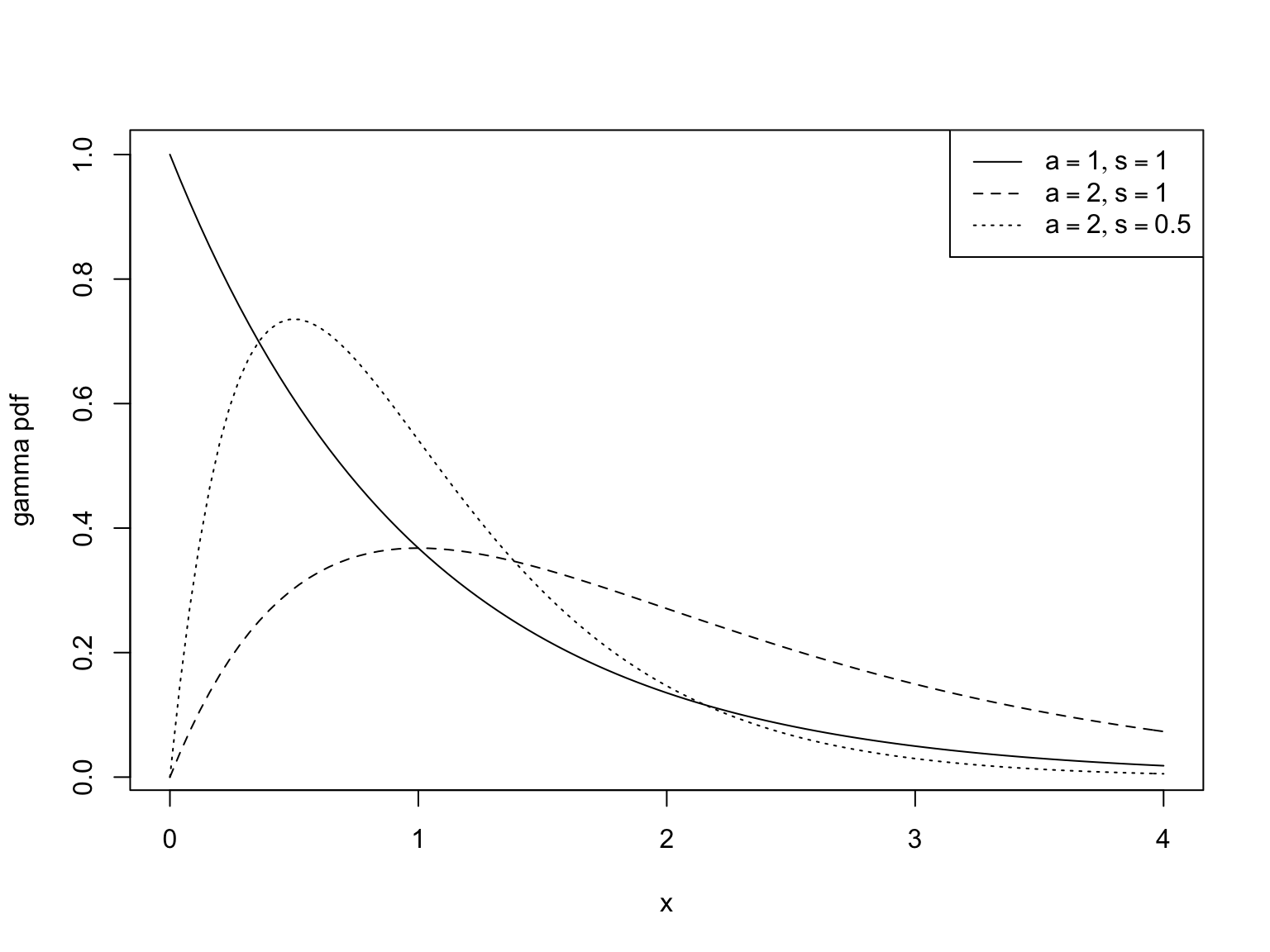# Fitting a parametric model (cont’d)

• Now maximize the likelihood
• For some (but not all) standard distributions, the function fitdistr in the package MASS has pre-programmed maximum likelihood estimates
• You saw this in homework 3 for the $$t$$ distribution
• As it happens, fitdistr knows about gamma distributions too
• It’s also easy to maximize the log-likelihood yourself
# Define the (negative, normalized) log-likelihood function
gamma.loglike <- function(params, data = cats$Bwt) { -mean(log(dgamma(x = data, shape = params, scale = params))) } # Ask R to optimize it, with a starting guess about the parameters cats.gamma <- optim(fn = gamma.loglike, par = c(1, 1)) # What is the best (negative, normalized) log-likelihood? cats.gamma$value
##  0.6677077
# What parameters did optim find for us?
cats.gamma$par ##  32.65187332 0.08341489 # Compare the fitted density to the histogram plot(hist(cats$Bwt, plot = FALSE, breaks = 20), freq = FALSE, xlab = "Body weight (kg)")
curve(dgamma(x, shape = cats.gamma$par, scale = cats.gamma$par), add = TRUE)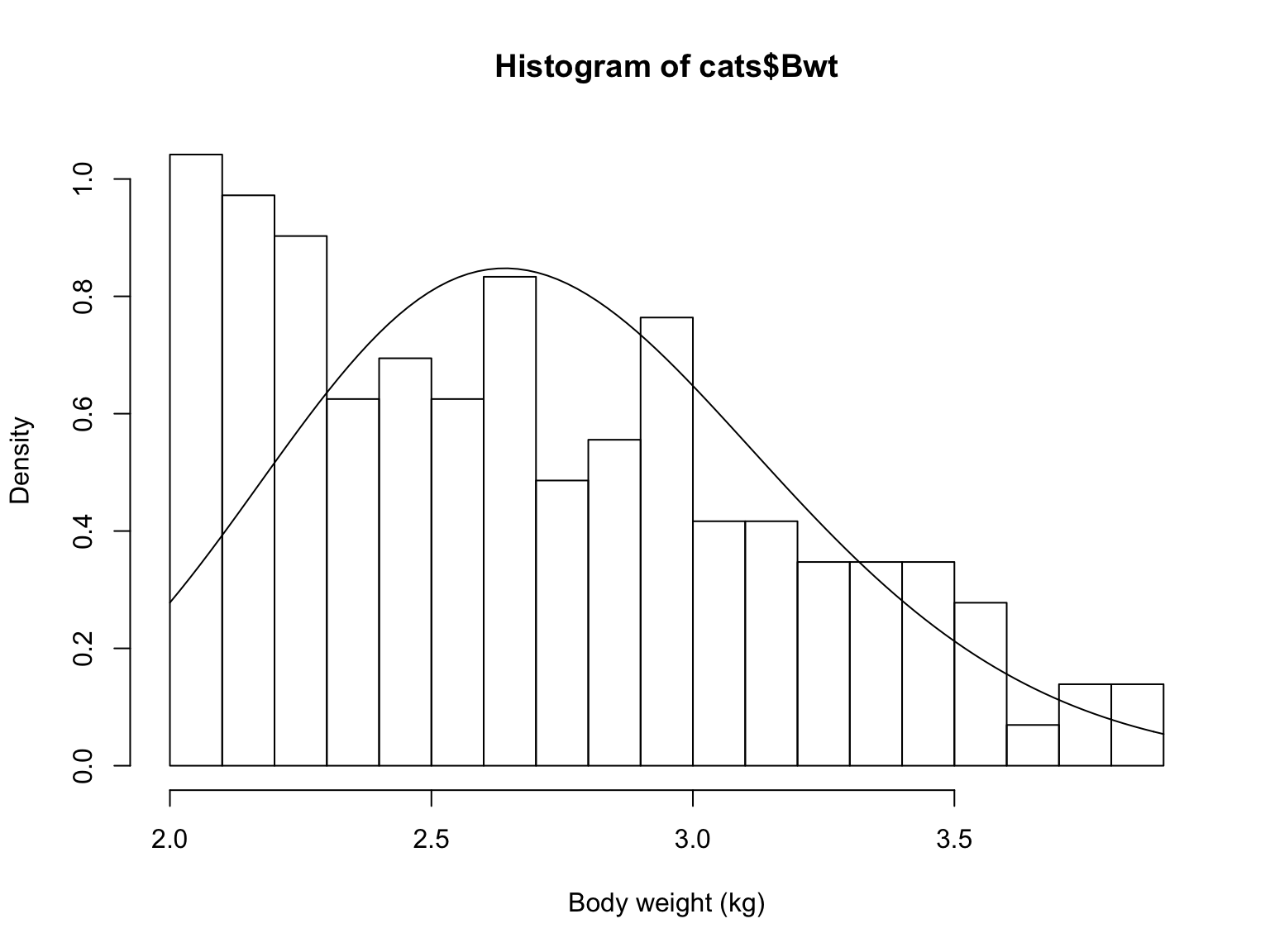# The histogram is already a density estimate

• Estimated density is constant on bins
• $$N_i =$$ number of samples in bin $$i$$
• $$i(x)=$$ which bin $$x$$ falls into
• $$h =$$ width of the bins

$\hat{f}_{hist}(x) \equiv \frac{1}{h} \frac{N_{i(x)}}{n}$

# Why this works

Probability of falling into a region of length $$h$$ around $$x$$ will be $\int_{x-h/2}^{x+h/2}{f(u) du} \approx f(x) h$ for very small $$h$$. So $N_i \sim \mathrm{Binom}(n, f(x) h)$ and $\hat{f}_{hist}(x) = \frac{N_i}{nh} \sim \frac{1}{nh} \mathrm{Binom}(n, f(x) h)$ which $$\rightarrow f(x)$$ as $$n \rightarrow \infty$$

# How bad is a histogram?

Error from variance: $$\propto 1/nh$$

Error from bias: $$\propto h^2 (f^{\prime}(x))^2$$

Total error for estimating the density, $$O(h^2) + O(1/nh)$$

Best $$h = O(n^{-1/3})$$

Best total error, $$O(n^{-2/3})$$

vs. $$O(n^{-1})$$ if we’ve got a correct parametric model

Q from discussion: What about variable-width bins?

A: That can help, but it’s even better to get away from fixed bins altogether

# Kernels

Idea: “Smooth out” each observation into a little distribution

Kernel functions are pdfs

Put a copy of the kernel at each observation; add them up

$\hat{f}_{kern}(x) = \frac{1}{n} \sum_{i=1}^{n}{\frac{1}{h} K\left(\frac{x-x_i}{h}\right)}$

# How this works for cats

Say $$h=0.01$$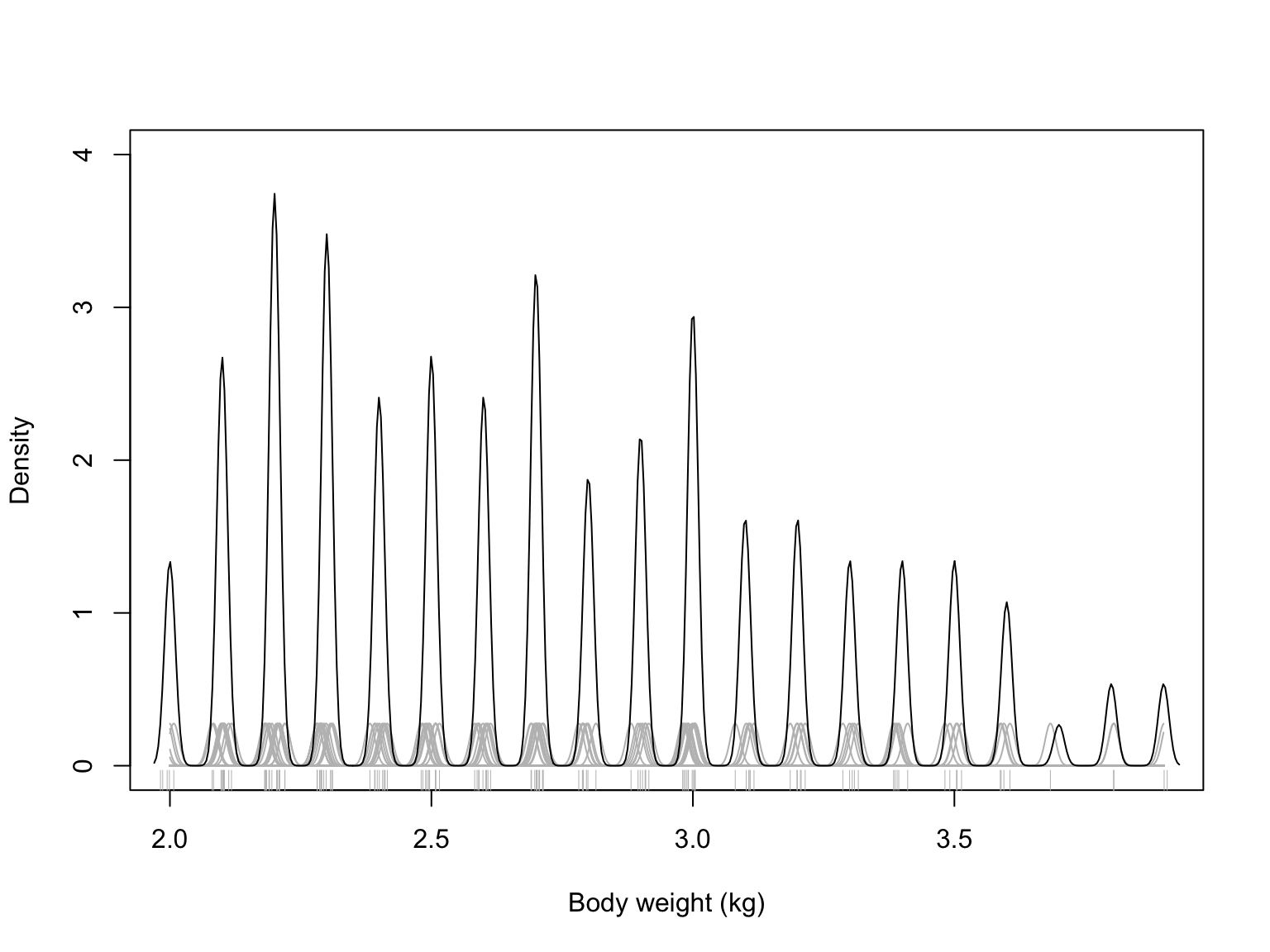# How do we pick the bandwidth?

• Cross-validation
• Cross-validate what?
• Integrated squared error: $$\int{(f(x) - \hat{f}(x))^2 dx}$$
• Or (negative, normalized) log-likelihood $$\int{-\log{(\hat{f}(x))} f(x) dx}$$

# Evaluating the log-likelihood

• The integral is an expected value: $\int{-\log{(\hat{f}(x))} f(x) dx} = \Expect{-\log{\hat{f}(X)}}$
• With $$n$$ IID samples $$x_i$$, $\Expect{-\log{\hat{f}(X)}} \approx \frac{1}{n}\sum_{i=1}^{n}{-\log{\hat{f}(x_i)}}$ by LLN
• A similar but more complicated trick for ISE; see backup slides

# Computationally: npudens

library(np)
bwt.np <- npudens(cats\$Bwt)
plot(bwt.np, xlab = "Body weight (kg)")